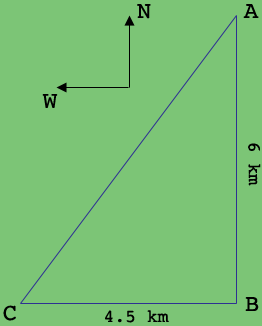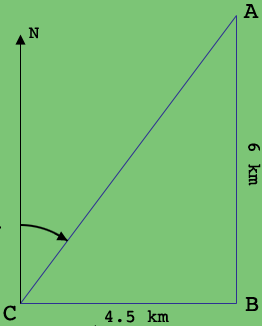SEARCH HOMEMath Central Quandaries & QueriesQuestion from Emma: A is 6km due north of turbine B C is 4.5km due west of turbine B Calculate the distance AC Then calculate the bearing of C from A Give your answer correct to the nearest degreeHi Emma,

I drew a diagram and marked north and west.The triangle $ABC$ is a right triangle so Pythagoras Theorem will give you the distance from $A$ to $C.$

In my second diagram I drew a line segment from $C$ pointing north. The bearing of $C$ to $A$ is the measure of the angle from north to $CA,$ measured clockwise from north. What trig function will help you determine the measure of this angle?PennyMath Central is supported by the University of Regina and The Pacific Institute for the Mathematical Sciences.